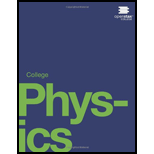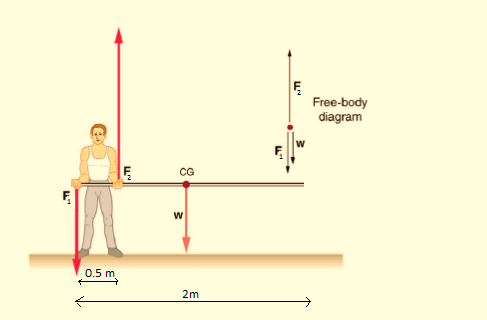# A person carries a plank of wood 2.00 m long with one hand pushing down on it at one end with a force F 1 and the other hand holding it up at .500 m from the end of the plank with force F 2 . If the plank has a mass of 20.0 kg and its center of gravity is at the middle of the plank, what are the magnitudes of the forces F 1 and F 2 ?### College Physics

1st Edition
Paul Peter Urone + 1 other
Publisher: OpenStax College
ISBN: 9781938168000

#### Solutions

Chapter
Section### College Physics

1st Edition
Paul Peter Urone + 1 other
Publisher: OpenStax College
ISBN: 9781938168000
Chapter 9, Problem 9PE
Textbook Problem
378 views

## A person carries a plank of wood 2.00 m long with one hand pushing down on it at one end with a force F1 and the other hand holding it up at .500 m from the end of the plank with force F2. If the plank has a mass of 20.0 kg and its center of gravity is at the middle of the plank, what are the magnitudes of the forces F1 and F2?

To determine

The magnitudes of the forces if a person F1 and F2 carries a plank of wood 2.00 m long with one hand pushing down on it at one end with a force F1  and the other hand holding it up at .500 m from the end of the plank with force F2 , the plank has a mass of 20.0 kg and its center of gravity is at the middle of the plank?### Explanation of Solution

Given:

Mass of plank = 20kg

Length of plank = 2.00 m

Distance between F1 and F2 = 0.5 m

Formula used:

Torque is given by the formula,

T=F×r , where F is the force and r is the perpendicular distance.

Weight, W = mg

Calculation:

For keeping the system in balance, the net torque at CG of plank must be zero.

Net torque in CG of plank

T = F1r1F2r2 =F1×1F2×0.5

Equating to zero,

F1×1F2×0.5=0F10

### Still sussing out bartleby?

Check out a sample textbook solution.

See a sample solution

#### The Solution to Your Study Problems

Bartleby provides explanations to thousands of textbook problems written by our experts, many with advanced degrees!

Get Started

Find more solutions based on key concepts
Give the molecular formula for each of the following structural formulas.

General Chemistry - Standalone book (MindTap Course List)

The length of time a person must spend exercising in order to meet the Physical Activity Guidelines for America...

Nutrition: Concepts and Controversies - Standalone book (MindTap Course List)

Why are titanium oxide features visible in the spectra of only the coolest stars?

Horizons: Exploring the Universe (MindTap Course List)

In the following diagram, designate each daughter cell as diploid (2n) or haploid (n).

Human Heredity: Principles and Issues (MindTap Course List)

A wire of nonmagnetic material, with radius R, carries current uniformly distributed over its cross section. Th...

Physics for Scientists and Engineers, Technology Update (No access codes included)

What happens in convergence zones? How is caballing associated with convergence zones?

Oceanography: An Invitation To Marine Science, Loose-leaf Versin